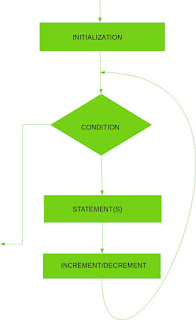# Learn For Loop In C

## Syntax:

```for (initialization;condition;increment/decrement)
{

statement(s);

}
```

### Flowchart:### Explantion:

Initialization: It means we have to set some staring values to the counter variable. This is same as initializing a normal variable.
For Example: i=0;

Condition: This is the terminating condition of the loop. If the expression written here evaluates to true then we say that the condition is met and for loop is terminated and control moves to the first statement outside the for loop. The condition depends on the purpose of the loop.

Increment or Decrement:This is where you increment or decrement the counter variable.
For Example: If you want to increment the values of counter variable i by 1, you can write it as i=i+1 or i++ (Post Increment) or ++i (Pre Increment). And if you want to decrease it by 1 the you can write i-- or i-- or i=i-1. You can increment or decrement the counter variable by any number you want.
For Example: i=i+i%2;

### Uses:

• Used to perform repeatative taks.

1. Initialization,condition and increment or decrement are grouped together which makes it easy for programmer to remember the things and simplifies the program.

### Problem:

Write a C program to print all even numbers between 1 to 10.

### Solution:

1. Use i as counter in for loop.
2. Initialize i=1,condition is i<=10 and set increment part as i++.
3. Check if i%2==0 means if i is divisible by 2 or not i.e even or not.
4. If even print the number else continue the next iteration.
```#include<stdio.h>

void main()
{

int i;

for(i=1;i<=10;i++)
{
if(i%2==0)
printf(" %d ",i);
}
}
```

### Explanation:

1. For the first iteration, i=1 then condition is checkedi.e i<=10 i.e 1<=10 which is true so the statements in the loop execute.
i%2 i.e 1%2=1. Hence i%2==0 is not true. So no number get printed.
2. Now after completion of one iteration 'i' is incremented.
NOTE: Counter is incremented only after completion of the iteration.
And counter variable is initialized ony for the first iteration after first iteration it is incremented or decremented.
So now i=2. This time 'i' is not initialized again. Condition is checked whether 2<=10 which is true. So the statements are executed again.
Now 2%2=0. Hence the statement i%2==o evaluates to true and 2 is printed.
Similarly remaining iterations get executed. And finally we get the output as given below:

```
2 4 6 8

```
If you have any doubts or suggestions for this tutorial please let men know in the comments so that I can correct it and others will get only the correct knowledge.